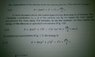# Conversion of energy expression from Cartesian to spherical coordinates

Syrus
A text I am reading displays the attached image. Can someone explain the general method for obtaining the velocity analogues of those terms (in parentheses) in 1.5? I know the second and third terms in parentheses in 1.6 and 1.7 are the squares of angular velocities, but can a general procedure be expressed to convert such equations between arbitrary coordinate frames?

In other words, how may we derive equation 1.6 (or 1.7) from 1.5 in an entirely mathematical sense?

(I am familiar with the coordinate transformations between Cartesian and spherical coordinates)

*In the attachment, a dot indicates over a symbol indicates the time-derivative of the quantity over which it appears.

#### Attachments

•IMAG0507.jpg
28 KB · Views: 381
Last edited: